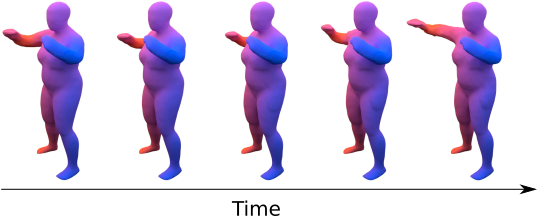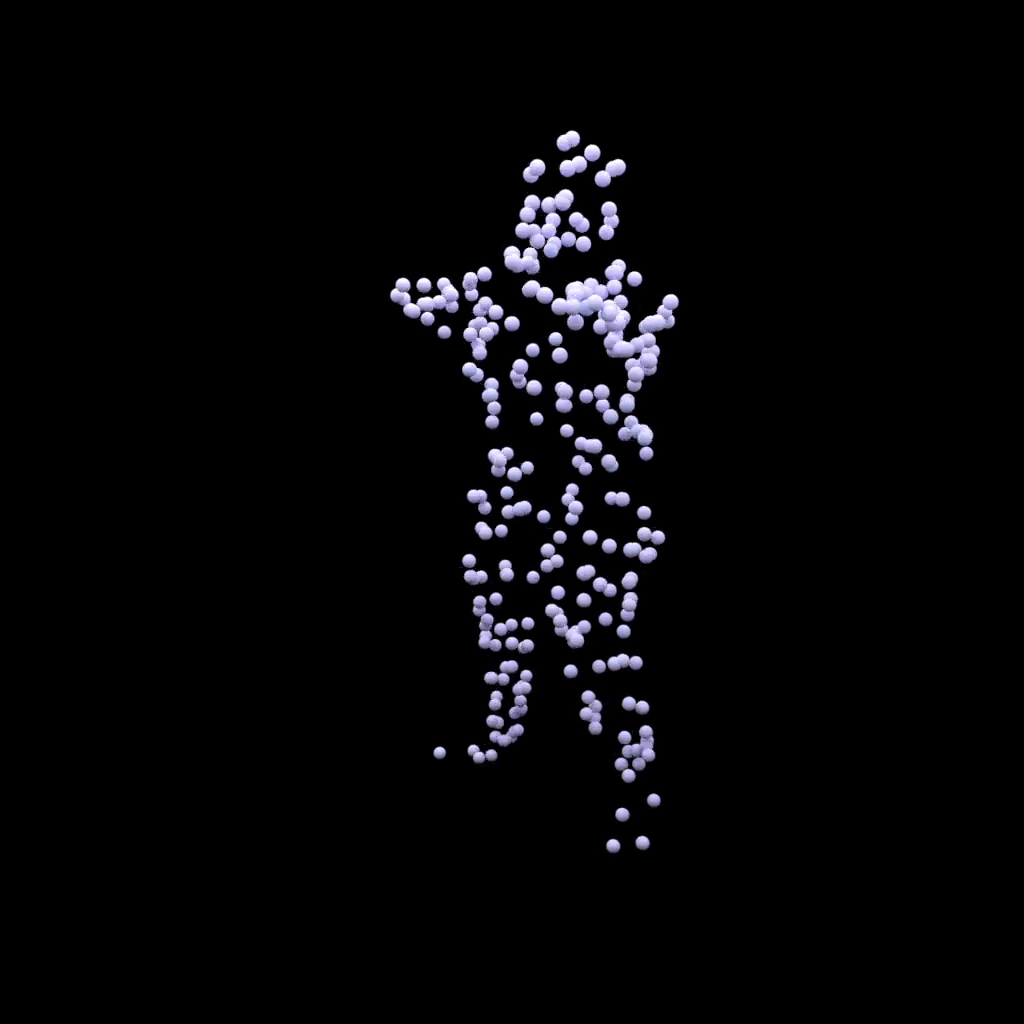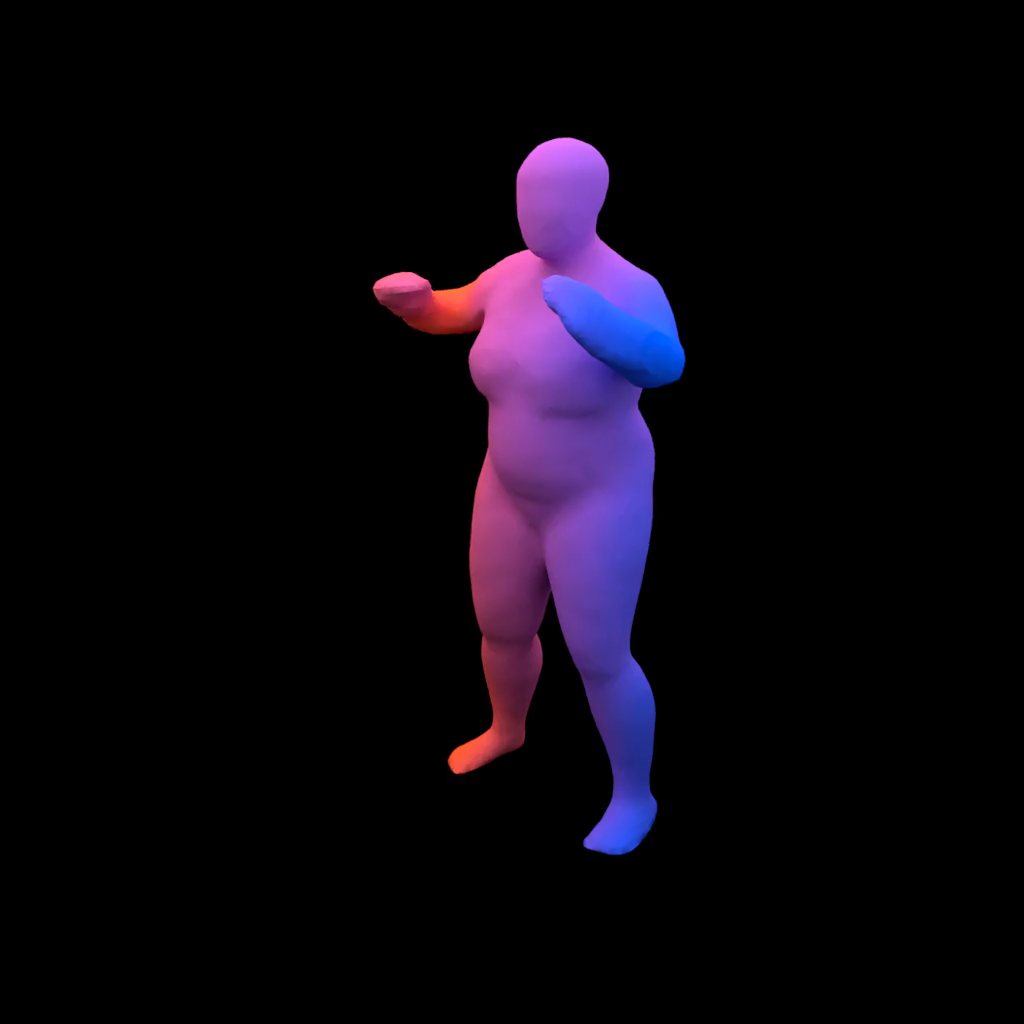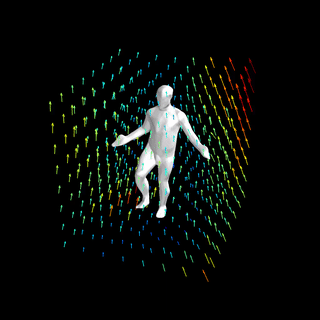# Occupancy FlowAn intelligent agent that interacts and navigates in our world has to be able to reason in 3D. Therefore, there has been a lot of interest in learning-based 3D reconstruction in recent years. Such techniques use neural networks to process some input information (for example an image or a sparse point cloud) and output the 3D geometry of a single object or scene.

One particular challenge in 3D reconstruction is the output representation, i.e. the representation of geometry, that we use. On the one hand, this representation should be expressive enough that we can represent all the complex geometries that appear in the real world. On the other hand, the representation should work well with modern machine learning techniques, in particular deep learning.

Recently, our group proposed a new output representation for learning-based 3D reconstruction, called Occupancy Networks. Occupancy Networks represent geometry through a deep neural network that distinguishes the inside from the outside of the object. We found that this representation is both powerful enough to represent complex geometries and also fits well the deep learning paradigm.

This allows us, for example, to train a neural network that can reconstruct a human from a sparse 3D point cloud:## The Challenge

While this works well, this scenario is not completely realistic: in the real world, objects are in motion, so that the human would not stand still, but move. Our input would hence rather look like this:Of course, we could treat each time step individually and apply our network to it. However, this would be very slow (after all we cannot reuse information from previous time steps) and even worse, we would not have any correspondences between the individual time steps. Where does the finger tip end up 2 seconds later? We can’t know as we are not able to identify where a specific point on the body (e.g. the tip of a finger) is at a later time step.

Can we do something more clever than this?

## Our Approach

Our key insight in this project is that we can represent shape and motion of the 3D body separately (“disentangled”). Instead of just using an occupancy network, we use two networks: an occupancy network and a velocity network. While the occupancy network represents the shape of the 3D object, the velocity network defines a motion field in 3D space that changes over time. We can visualize this as follows:During inference, we can extract a mesh at time $t=0$ using our occupancy network (like in our previous paper) and then propagate the vertices forward in time using the velocity network by solving an ordinary differential equation.

During training, we sample random points in 3D and go backward in time to determine where these points would have been at $t=0$. We then evaluate the occupancy network at these hypothetical locations and compare to the ground truth occupancy at $t=\tau$. This way we do not even need correspondences between different time steps in our training data! However, if they are given, we can easily incorporate them by comparing the location where a point at $t=0$ ends up at $t=\tau$ following our velocity network with the location where it should go.

All in all, the key idea is that we disentangle the shape and the motion of 3D objects. As we use our continuous velocity network for the motion representation, we automatically have correspondences for every point in space between time steps. This also makes the inference step much faster!

## Experiments

Let’s look at our example from the introduction again. How does Occupancy Flow perform on this example? Here are the results:We see that Occupancy Flow reconstructs the 3D motion in a plausible way and also got the correspondences right (indicated by the colors).

In our paper, we conducted a variety of other experiments. For example, we used a generative model to transfer the motion from one shape to another:## Further Information

You can find more information (including the paper and supplementary) on our project page. We also provide animated slides for our project. If you are interested in experimenting with Occupancy Flow yourself, download the source code of our project and run the examples. We are happy to receive your feedback!

@inproceedings{Niemeyer2019ICCV,
title = {Occupancy Flow: 4D Reconstruction by Learning Particle Dynamics},
author = {Niemeyer, Michael and Mescheder, Lars and Oechsle, Michael and Geiger, Andreas},
booktitle = {International Conference on Computer Vision (ICCV)},
year = {2019},
}


Tags:

Categories:

Updated: